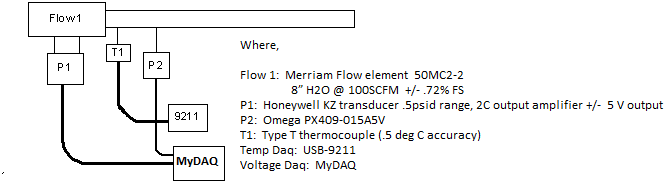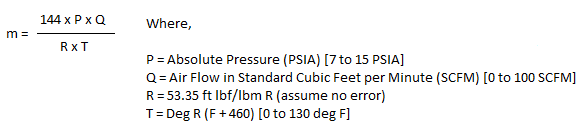# Curriculum and Labs for Engineering Education

cancel
Showing results for
Did you mean:

## ME Lab 5 - Perturbation

Procedure:

Calculate the uncertainty of the mass flow reading determined by the data acquisition in the following diagram.Your base calculation should represent the maximum air flow that the system is capable of measuring.1.    For each variable in the flow equation, list the variable, and each of the perturbations that will be conducted on it.  If you have expanded the equation to include the additional levels, include your new expanded formula.  If you chose to equate the lower levels to upper levels, show how that was done.
2.    Show the  calculation  for determining σ+ and σ- for the pressure reading data acquisition error.
3.    Calculate σ+ and σ- for each of the listed variables and combinations.  You may do this long hand or with a spreadsheet.  Put the answers in a table form showing the variable and what is being pertubated (such as pressure transducer linearity).  Include your σ+ and σ- in this table.
4.    Use your math program with these values to calculate the total  uncertainty in the mass flow value using column A for the nominal value, column B for σ+ and column C for σ-.  Enter the input matrix for your calculation and print the front panel for the program  showing the full input and output arrays.

Report and Submission:

Present your work in a memo format for grading.  You may work in teams  of not more than two people.  Make all your work legible.  Illegible  work will not be graded for any partial credit.